MODEL Statement
MODEL fitting-options </ model-options> ;

You specify the MODEL statement if you want to fit a theoretical semivariogram model to the empirical semivariogram data that are produced in the COMPUTE statement. You must have nonmissing empirical semivariogram estimates at a minimum of three lags to perform model fitting.

You can choose to perform a fully automated fitting or to fit one model with specific forms. In the first case you simply specify a list of forms or no forms at all. All suitable combinations are tested, and the result is the model that produces the best fit according to specified criteria. In the second case you specify one theoretical semivariogram model, and you have more control over its parameters for the fitting process.

Furthermore, you can specify a theoretical semivariogram model in two ways:

• You explicitly specify the FORM option and any of the options SCALE, RANGE, and NUGGET in the MODEL statement.

• You can specify an MDATA= data set. This data set contains variables that correspond to the FORM option and to any of the options SCALE, RANGE, NUGGET, and SMOOTH. You can also use an MDATA= data set to request a fully automated fitting.

The two methods are exclusive; either you specify all parameters explicitly, or they all are read from the MDATA= data set.

The MODEL statement has the following fitting-options:

ALPHA=number

requests that a t-type confidence interval be constructed for each of the fitting parameters with confidence levelnumber. The value of number must be in; the default is 0.05 which corresponds to the default confidence level of 95%. If the CL option of the MODEL statement is not specified, then ALPHA= is ignored.

CHOOSE=criterion
CHOOSE=(criterion1 ...criterionk)

specifies that if the fitting task has more than one model to fit, then PROC VARIOGRAM ranks the fitted models and chooses the optimally fit model according to one or more available criteria.

If you want to use multiple fitting criteria, then the order in which you specify them in the CHOOSE= option defines how they are applied. This feature is useful when fitting suggests that two or more models perform equally well according to a certain criterion. For example, if two models are equivalent according to the current criterion, then they are further ranked in the list based on the following criterion.

Each criterion can be one of the following:

AIC

specifies Akaike’s information criterion.

SSE

specifies the weighted sum of squares error for each fitted model when METHOD=WLS, and the residual sum of squares error for each fitted model when METHOD=OLS.

STATUS

classifies models based on their fitting process convergence status. CHOOSE=STATUS places on top models for which the fitting process is successful.

By default, the models are ranked in the fit summary table with the best fitted model at the top of the list, based on the criteria that you specify in the CHOOSE= option. This model is the fit choice of PROC VARIOGRAM for the particular fitting task. If you omit the CHOOSE= option, then the default behavior is CHOOSE=(SSE AIC).

Regardless of the specified fitting criteria, models for which the fitting process is unsuccessful always appear at the bottom of the fit summary table. For more details about the fitting criteria, see the section Fitting Criteria. After multiple models are ranked, they are further categorized in classes of equivalence depending on whether any two models calculate the same semivariance value at the same distance for a series of different distances. For more details, see the section Classes of Equivalence.

If you specify the same criterion multiple times in the CHOOSE= option, then only the first instance is used for the ranking process and any additional ones are ignored. If you specify only one model to fit in the MODEL statement and you specify the CHOOSE= option, then the option is ignored.

CL

requests that t-type confidence limits be constructed for each of the fitting parameters estimates. The confidence level is 0.95 by default; this can be changed with the ALPHA= option of the MODEL statement.

EQUIVTOL=etol-value
ETOL=etol-value

specifies a positive upper value tolerance to use when categorizing multiple models in classes of equivalence. For this categorization, the VARIOGRAM procedure computes the sum of absolute differences of semivariances for pairs of consecutively ranked models. If the sum is lower than the EQUIVTOL= value for any such model pair, then these two models are deemed to be equivalent. As a result, the EQUIVTOL= option can affect the number and size of classes of equivalence in the fit summary table. Smaller values of the EQUIVTOL= parameter result in a more strict model comparison and can lead to a higher number of classes of equivalence. For more details, see the section Classes of Equivalence.

The default value for the EQUIVTOL= parameter is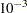. The EQUIVTOL= option applies when you fit multiple models with the FORM=AUTO option of the MODEL statement; otherwise, it is ignored.

The EQUIVTOL= option is independent of the ranking results from the RANKEPS= option of the MODEL statement. This means that you could possibly have models listed but not ranked in the fit summary table, and still have equivalence classes assigned according to the order in which the models appear in the table.

FIT=fit-type-options

specifies which type of empirical semivariogram to fit. You can choose between the following fit-type-options:

CLASSICAL
CLA

fits a model for the classical empirical semivariance.

ROBUST
ROB

fits a model for the robust empirical semivariance. This option can be used only when the ROBUST option is specified in the COMPUTE statement.

The default value is FIT=CLASSICAL.

FORM=form
FORM=(form1, ..., formk)
FORM=AUTO (auto-options)

specifies the functional form (type) of the semivariogram model. The supported structures are two-parameter models that use the sill and range as parameters. The Matérn model is an exception that makes use of a third smoothing parameter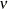.

The FORM= option is required when you specify the MODEL statement. You can perform fitting of a theoretical semivariogram model either explicitly or in an automated manner. For the explicit specification you specify suitable model forms in the FORM= option. For an automated fit you specify the FORM=AUTO option which has the AUTO(MLIST=) and AUTO(NEST=) suboptions. You can read more details in the following two subsections.

### Explicit Model Specification

You can explicitly specify a theoretical semivariogram model to fit by using any combination of one, two, or three forms. Use the syntax with the single form to specify a non-nested model. Use the syntax withstructures formi,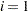, ...,, to specify up to three nested structures (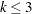) in a semivariogram model. Each of the forms can be any of the following:

CUBIC | EXPONENTIAL | GAUSSIAN | MATERN |
PENTASPHERICAL | POWER | SINEHOLEEFFECT | SPHERICAL
CUB | EXP | GAU | MAT | PEN | POW | SHE | SPH

All of these forms are presented in more detail in the section Theoretical Semivariogram Models. In addition, you can optionally specify a nugget effect for your model with the NUGGET option in the MODEL statement.

For example, the syntax

```FORM=GAU
```

specifies a model with a single Gaussian structure. Also, the syntax

```FORM=(EXP,SHE,MAT)
```

specifies a nested model with an exponential, a sine hole effect, and a Matérn structure. Finally

```FORM=(EXP,EXP)
```

specifies a nested model with two structures both of which are exponential.

Note: In the documentation, models are named either by using their full names or by using the first three letters of their structures. Also, the names of different structures in a nested model are separated by a hyphen (-). According to this convention, the previous examples illustrate how to specify a GAU, an EXP-SHE-MAT, and an EXP-EXP model, respectively, with the FORM= option.

When you explicitly specify the types of structures, you can fix parameter values or ask PROC VARIOGRAM to select default initial values for the forms parameters by using the SCALE, RANGE, NUGGET, and SMOOTH options. You can set your own, non-default initial parameter values by using the PARMS statement in combination with an explicitly specified semivariogram model in the MODEL statement.

### Automated Model Selection

Use the FORM=AUTO option to request the highest level of automation in the best fit selection of the parameters. If you specify FORM=AUTO, any of the SCALE, RANGE, or SMOOTH options that are also specified are ignored. When you specify the FORM=AUTO option, you cannot specify the PARMS statement for the corresponding MODEL statement. As a result, when you use the FORM=AUTO option, you cannot fix any of the model parameters and PROC VARIOGRAM sets initial values for them.

The AUTO option has the following auto-options:
MLIST=mform
MLIST=(mform1, ..., mformp)

specifies one or more different model forms to use in combinations during the model fitting process. If you omit the MLIST= suboption, then combinations are made among all available model types. The mform can be any of the following eight forms:

CUBIC | EXPONENTIAL | GAUSSIAN | MATERN |
PENTASPHERICAL | POWER | SINEHOLEEFFECT | SPHERICAL
CUB | EXP | GAU | MAT | PEN | POW | SHE | SPH

If you use more than one mform, then each mformi,, ...,must be different from the others in the group of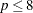forms that you specify.

NEST=nest-list

specifies the number of nested structures to use for the fitting. You can choose between the following to specify the nest-list:a single valueTOa sequence in whichequals the starting value andequals the ending value

For example,

```   NEST=1
```

produces the best fit with one single model among all model types specified in the MLIST= suboption. Also,

```   NEST=2 TO 3
```

produces the best fit among all combinations of the model types specified in the MLIST= suboption that result in nested models with two or three structures. The combinations that are tested include repetitions. Hence, if you specify, for example,

```   MODEL FORM=AUTO(MLIST=(EXP,SPH) NEST=1 TO 2)
```

then the different models that are tested are equivalent to the specifications FORM=EXP, FORM=SPH, FORM=(EXP,EXP), FORM=(EXP,SPH), FORM=(SPH,SPH) and FORM=(SPH,EXP). Note: The models EXP-SPH and SPH-EXP are taken as two separate models. Although they are mathematically equivalent (see the section Nested Models), PROC VARIOGRAM assigns different initial values to the model structures in each case, which can lead to different fitting results. (See the section Aspects of Semivariogram Model Fitting.)

If you omit the NEST suboption, then by default PROC VARIOGRAM searches for the best fit with up to three nested structures in a model. The default behavior is equivalent to

```   NEST=1 TO 3
```

In the VARIOGRAM procedure you can use a maximum of three nested structures to fit an empirical semivariogram; that is,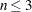.

You can use the AUTO value for the form in the MDATA= data set, and also in the FORM= option. However, in the former case the automation functionality is limited compared to the latter case and the auto-options of the FORM=AUTO option. In particular, when you specify the form to be AUTO in the MDATA= data set, then PROC VARIOGRAM follows only the default behavior and searches among all available forms for the best fit with up to three nested structures in a model.

MDATA=SAS-data-set

specifies the input data set that contains parameter values for the covariance or semivariogram model. The MDATA= data set must contain a variable named FORM, and it can optionally include any of the variables SCALE, RANGE, NUGGET, and SMOOTH.

The FORM variable must be a character variable. It accepts only the AUTO value or the form values that can be specified in the FORM= option in the MODEL statement. The RANGE, SCALE, NUGGET, and SMOOTH variables must be numeric or missing.

The number of observations present in the MDATA= data set corresponds to the level of nesting of the semivariogram model. Each observation line describes a structure of the model you submit for fitting.

If you specify the AUTO value for the FORM variable in an observation, then you cannot specify additional nested structures in the same data set, and any parameters you specify in the same structure are ignored. In that case, PROC VARIOGRAM performs a crude automated search among all available forms to obtain the best fit with up to three nested structures in a model. You can refine this type of search with additional suboptions when you perform it with the FORM=AUTO option instead of the MDATA= option in the MODEL statement.

When you have a nested model, you might want to specify parameter values for only some of the nested structures. In this case, you must specify the corresponding parameter values for the remaining model structures as missing values.

For example, you can use the following DATA step to specify a non-nested model that uses a spherical covariance within an MDATA= data set:

```data md1;
input scale range form \$;
datalines;
25 10 SPH
run;
```

Then, you can use the md1 data in the MODEL statement of PROC VARIOGRAM as shown in the following statements:

```proc variogram data=...;
compute ...;
model mdata=md1;
run;
```

This is equivalent to the following explicit specification of the semivariance model parameters:

```proc variogram data=...;
compute ...;
model form=sph scale=25 range=10;
run;
```

The following data set md2 is an example of a nested model:

```data md2;
input form \$ scale range nugget smooth;
datalines;
SPH 20 8 5 .
MAT 12 3 5 0.7
GAU .  1 5 .
;
```

This specification is equivalent to the following explicit specification of the semivariance model parameters:

```proc variogram data=...;
compute ....;
model form=(sph,mat,gau)
scale=(20,12,.) range=(8,3,1) smooth=0.7 nugget=5;
run;
```

Use the SMOOTH variable column in the MDATA= data set to specify the smoothing parameterin the Matérn semivariogram models. The SMOOTH variable values must be positive and no greater than 1,000,000. PROC VARIOGRAM sets this upper limit for numerical and performance reasons. In any case, if the fitting process leads the smoothness value to exceed the default threshold value 10,000, then the VARIOGRAM procedure converts the Matérn form into a Gaussian form and repeats the model fitting. To adjust the switching threshold value, you can use the MTOGTOL= option in the MODEL statement.

If you specify a SMOOTH column in the MDATA= data set, then its elements are ignored except for the rows in which the corresponding FORM is Matérn.

The NUGGET variable value is the same for all nested structures. This is the way to specify a nugget effect in the MDATA= data set. If you specify more than one nugget value for different structures, then the last nugget value specified is used.

METHOD=method-options

must be specified in the MODEL statement to fit a theoretical model to the empirical semivariance. The METHOD option has the following suboptions:

OLS

specifies that ordinary least squares be used for the fitting.

WLS

specifies that weighted least squares be used for the fitting.

The default is METHOD=WLS.

NEPSILON=min-nugget-factor
NEPS=min-nugget-factor

specifies that a minimal nugget effect be added to the theoretical semivariance in the unlikely occasion that the theoretical semivariance becomes zero during fitting with weighted least squares. As explained in the section Theoretical and Computational Details of the Semivariogram, the theoretical semivariance is always positive for any distance larger than zero. If a conflicting situation emerges as a result of numerical fitting issues, then the NEPSILON= option can help you alleviate the problem by adding a minimal variance at the distance lag where the issue is encountered. For more details, see the section Parameter Initialization.

If you omit the NEPSILON= option, then PROC VARIOGRAM sets a default value of. If a minimal nugget effect is used, its value is case-specific and is based on the min-nugget-factor. Specifically, its value is defined as min-nugget-factor times the sample variance of the input data set, or as min-nugget-factor when the sample variance is equal to zero.

NUGGET=number

specifies the nugget effect for the model. The nugget effect is due to a discontinuity in the semivariogram as determined by plotting the sample semivariogram; see Theoretical Semivariogram Models for more details. The NUGGET= parameter is a nonnegative number. If you specify a nonmissing value, then it is used as a fixed parameter in the fitting process.

PROC VARIOGRAM assigns a default initial value for the nugget effect in the following cases:

• if you specify a missing value.

• if you omit the NUGGET= option and you do not specify an associated PARMS statement with initial values for the nugget.

The NUGGET= option is incompatible with the specification of the PARMS statement for the corresponding MODEL statement.

RANGE=range
RANGE=(range1, ..., rangek)

specifies the range parameter in semivariogram models. The RANGE= option is optional. However, if you specify the RANGE= option, then you must provide range values for all structures that you have specified explicitly in the FORM= option. All nonmissing range values are considered as fixed parameters. PROC VARIOGRAM assigns a default initial value to any of the model structures for which you specify a missing range value. PROC VARIOGRAM assigns default initial values to all model structures if you omit the RANGE= option, unless you specify an associated PARMS statement and initial values for the range in it.

The range parameter is a positive number, has the units of distance, and is related to the correlation scale of the underlying spatial process.

Note: If you specify this parameter for a power model, then it does not correspond to a range. For power models, the parameter you specify in the RANGE option is a dimensionless power exponent whose value must range within [0,2) so that the power model is a valid semivariance function.

The RANGE= option is ignored when you specify the FORM=AUTO option. The RANGE= option is incompatible with the specification of the PARMS statement for the corresponding MODEL statement.

RANGELAG=rlag-list
RLAG=rlag-list

specifies that you prefer to use the range of consecutive nonmissing empirical semivariance lags in the rlag-list for the semivariogram fitting process, instead of using all MAXLAGS1 lag classes by default. You can specify rlag-list in either of the following forms:a single value that designates the width of the selected lag range by starting at lag zero. You must use at least three lags to perform model fitting, so you can specifywithin [3,, MAXLAGS1].TOa sequence in whichequals the starting lag andequals the ending lag. The parametersandmust be nonnegative integer numbers to designate lag classes between zero and MAXLAGS. Use at least three lags for model fitting; hence it holds that.

The following two brief examples exhibit the use of the RANGELAG option. These examples assume that you have set the MAXLAGS= option to 9 or higher to indicate nonmissing empirical semivariance estimates at 10 lags or more.

In the first example,

```RANGELAG=8
```

uses the empirical semivariance in the first eight lags to fit a theoretical model. Hence, RANGELAG=8 uses only the lag classes zero to seven. This approach enables you to account only for the correlation behavior described by the firstempirical semivariogram lag classes.

In the second example,

```RANGELAG=2 TO 9
```

specifies that the empirical semivariance values at lag classes zero, one, and after lag class nine are excluded from the model fitting process.

RANKEPS=reps-value
REPS=reps-value

specifies the minimum threshold to compare fit quality of two models for a specific criterion. Beyond this threshold the criterion values become insensitive to comparison. In particular, when you fit multiple models, PROC VARIOGRAM computes for each one the value of the fitting criterion specified in the CHOOSE= option of the MODEL statement. These values are examined in pairs at the sorting stage. If the difference of a given pair exceeds the reps-value, then the sorting order of the corresponding models is reversed; otherwise, the two models retain their relative order in the rankings. Hence, the RANKEPS= option can affect model ranking in the fit summary table.

The default value for the RANKEPS= parameter isand accounts for the default optimization convergence tolerance at the fitting stage prior to model ranking. The convergence tolerance itself limits the accuracy that you can use to compare two models under a given criterion. As a result, smaller values of the RANKEPS= parameter might not lead to a sensible and more strict model comparison because for a smaller reps-value, ranking could depend on digits beyond the accuracy limit.

In the opposite end, if the specified reps-value turns out to be large compared to the criterion value differences, then it can make the sorting process insensitive to the specified sorting criterion. When this happens, the fit summary table ranking reflects only the order in which different models are examined in the procedure flow. You can tell whether the criterion is bypassed; if it is, then one or more values of the specified criterion might not appear to be sorted in the fit summary table.

The RANKEPS= parameter must be a positive number. The RANKEPS= option applies when you fit multiple models with the FORM=AUTO option of the MODEL statement; otherwise, it is ignored.

SCALE=scale
SCALE=(scale1, ..., scalek)

specifies the scale parameter in semivariogram models. The SCALE= option is optional. However, if you specify the SCALE= option, then you must provide sill values for all structures that you have specified explicitly in the FORM= option. All nonmissing scale values are considered as fixed parameters. PROC VARIOGRAM assigns a default initial value to any of the model structures for which you specify a missing scale value. PROC VARIOGRAM assigns default initial values to all model structures if you omit the SCALE= option, unless you specify an associated PARMS statement with initial values for scale.

The scale parameter is a positive number. It has the same units as the variance of the variable in the VAR statement. The scale of each structure in a semivariogram model represents the variance contribution of the structure to the total model variance.

In power models the SCALE= parameter does not correspond to a sill because the power model has no sill. Instead, PROC VARIOGRAM uses the SCALE= option to designate the slope (or scaling factor) in power model forms. The power model slope has the same variance units as the variable in the VAR statement.

The SCALE= option is ignored when you specify the FORM=AUTO option. The SCALE= option is incompatible with the specification of the PARMS statement for the corresponding MODEL statement.

SMOOTH=smooth
SMOOTH=(smooth1, ..., smoothm)

specifies the positive smoothness parameterin the Matérn type of semivariance structures. The special caseis equivalent to the exponential model, whereas the theoretical limit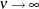gives the Gaussian model.

The SMOOTH= option is optional. When you specify an explicit model in the FORM= option withMatérn structures, you can provide up tosmoothness values. You can specify a value for smoothi,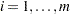that is positive and no greater than 1,000,000. PROC VARIOGRAM sets this upper limit for the SMOOTH= option values for numerical and performance reasons. In any case, if the fitting process leads the smoothness value to exceed the default threshold value 10,000, then the VARIOGRAM procedure converts the Matérn form into a Gaussian form and repeats the model fitting. To adjust the switching threshold value, you can use the MTOGTOL= option in the MODEL statement.

If you specify fewer thanvalues, then the remaining Matérn structures have their smoothness parameters initialized to missing values. If you specify more thanvalues, then values in excess are ignored.

All nonmissing smoothness values are considered as fixed parameters of the corresponding Matérn structures. PROC VARIOGRAM assigns a default initial value to any of the model Matérn structures, if any, for which you specify a missing smoothness value. PROC VARIOGRAM assigns default initial values to all model Matérn structures if you omit the SMOOTH= option, unless you specify an associated PARMS statement and initial values for smoothness in it.

The SMOOTH= option is ignored when you specify the FORM=AUTO option. The SMOOTH= option is incompatible with the specification of the PARMS statement for the corresponding MODEL statement.

In addition to the fitting-options, you can specify the following model-options after a slash (/) in the MODEL statement.

COVB

requests the approximate covariance matrix for the parameter estimates of the model fitting. The COVB option is ignored when you also specify the DETAILS=ALL option.

When you specify an explicit model with the FORM= option in the MODEL statement, the COVB option produces the requested approximate covariance matrix. When you specify the FORM=AUTO option in the MODEL statement, by default the COVB option produces output only for the selected model, where the choice is based on the criteria that you specify in the CHOOSE= option of the MODEL statement. If you specify the DETAILS option in addition to FORM=AUTO in the MODEL statement, then the COVB option produces output for each one of the fitted models.

CORRB

requests the approximate correlation matrix for the parameter estimates of the model fitting. The CORRB option is ignored when you also specify the DETAILS=ALL option.

When you specify an explicit model with the FORM= option in the MODEL statement, the CORRB option produces the requested approximate correlation matrix. When you specify the FORM=AUTO option in the MODEL statement, by default the CORRB option produces output only for the selected model, where the choice is based on the criteria that you specify in the CHOOSE= option of the MODEL statement. If you specify the DETAILS option in addition to FORM=AUTO in the MODEL statement, then the CORRB option produces output for each one of the fitted models.

DETAILS <= detail-level>

requests different levels of output to be produced during the fitting process. You can specify any of the following detail-level arguments:

MOD

specifies that the default output for all candidate models be produced when the FORM=AUTO option is specified in the MODEL statement. If you fit only one explicit model, then the DETAILS=MOD option has no effect and is ignored.

ITR

requests that a complete iteration history be produced in addition to the default output. The output for DETAILS=ITR includes the current values of the parameter estimates, their gradients, and additional optimization statistics.

ALL

requests the most detailed level of output when fitting a model. Specifically, except for the default output, the DETAILS=ALL option produces optimization statistics in addition to the combined output of the DETAILS=ITR, COVB, and CORRB options.

When you fit multiple models with the FORM=AUTO option in the MODEL statement, only the selected model default output is produced. The model selection is based on the criteria that you specify in the CHOOSE= option of the MODEL statement. With the DETAILS option you can produce ODS tables with information about the fitting process of all the models that you fit. Moreover, you can produce output at different levels of detail that you can specify with the detail-level argument.

Omitting the DETAILS option or specifying the DETAILS option without any argument is equivalent to specifying DETAILS=MOD.

displays the gradient of the objective function with respect to the parameter estimates in the "Parameter Estimates" table.

MTOGTOL=number
MTOL=number

specifies a threshold value for the smoothness parameter of the Matérn form. Above this threshold, a Matérn form in a model switches to the Gaussian form. The number value must be positive and no greater than 1,000,000, which is the smoothness upper bound set by the VARIOGRAM procedure.

By default, if the fitting process progressively increases the Matérn smoothness parameterwithout converging to a smoothness estimate, then PROC VARIOGRAM converts the Matérn form into a Gaussian form when smoothness exceeds the default value 10,000. If you specify the number value to be greater than the 1,000,000 boundary value, then it is ignored and reset to the default threshold value. For more details about the Matérn-to-Gaussian form conversion, see the section Fitting with Matérn Forms.

NOFIT

suppresses the model fitting process.

NOITPRINT

suppresses the display of the iteration history table when you have also specified the DETAILS=ITR or DETAILS=ALL option in the MODEL statement. Otherwise, the NOITPRINT option is ignored.